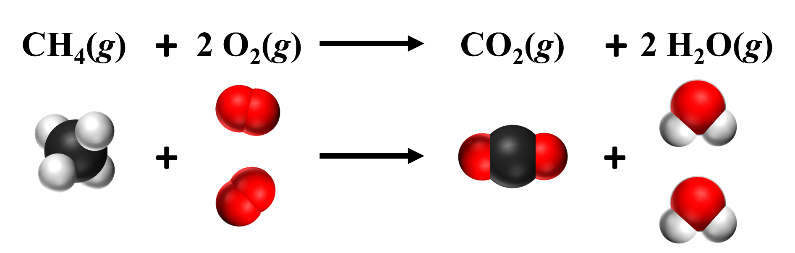# 2.1: Balancing Chemical Reactions

Part A – The Basics

According to the Law of Conservation of Matter, matter can neither be created nor destroyed. This means that in any chemical reaction, any atoms that are present in the reactants should also be present in the products. Subscripts in a chemical formula indicate how many of each atom is present. If there is a subscript outside of a parenthesis, it should be multiplied by all the subscripts within the parentheses:For example, in the compound above there are 2 aluminum atoms, 3 sulfur atoms and 12 oxygen atoms.

Example $$\PageIndex{1}$$

Identify and count the atoms of both the reactants and products in the following unbalanced chemical equation.

C2H6 + O2 → CO2 + H2O

Solution

 Atom Number in Reactants Number in Products C 2 (in C2H6) 1 (in CO2) H 6 (in C2H6) 2 (in H2O) O 2 (in O2) 3 (2 in CO2 + 1 in H2O)

Example $$\PageIndex{2}$$

Identify and count the atoms of both the reactants and products in the following unbalanced reaction.

Al + H2SO4 → Al2(SO4)3 + H2

Solution

 Atom Number in Reactants Number in Products Al 1 (in Al) 2 (in Al2SO4) H 2 (in H2SO4) 2 (in H2) S 1 (in H2SO4) 3 (in Al2(SO4)3) O 4 (in H2SO4) 12 (in Al2(SO4)3)

Exercise $$\PageIndex{1}$$

Identify and count the atoms in both the reactants and products in the following reactions.

a) Al + HCl → AlCl3 + H2

b) Pb(NO3)2 + NaCl → PbCl2 + NaNO3

 Atom Number in Reactants Number in Products Al 1 (in Al) 1 (in AlCl3) H 1 (in HCl) 2 (in H2) Cl 1 (in HCl) 1 (in AlCl3)

 Atom Number in Reactants Number in Products Pb 1 (in Pb(NO3)2 1 (in PbCl2) N 2 (in Pb(NO3)2) 2 (in NaNO3) O 6 (in Pb(NO3)2) 3 (in NaNO3) Na 1 (in NaCl) 1 (in NaNO3) Cl 1 (in NaCl) 2 (in PbCl2)

Part B – Adding coefficients to balance reactions

To balance a reaction means to make the number of atoms the same on both the reactants and products side. To do so, coefficients need to be added to the chemical equation. Coefficients are whole numbers that are placed in front of the element or compound in the equation to indicate how many units of each substance participate in the chemical reaction.

When counting atoms, coefficients in front of a molecule should be multiplied by the subscripts of all atoms in the molecule:In the reaction below, a ‘2’ was placed in front of both the O2 and the H2O to balance the reaction. This means that there are two O2 molecules and two H2O molecules, as shown in the diagram below. This balances the atoms, making the number of atoms the same on both the reactants and products side. When balancing chemical reactions, you can only change the coefficients – never change the subscripts. Changing the subscripts changes the identity of the molecule. Coefficients of ‘1’ are omitted.Figure $$\PageIndex {1}$$: One methane molecule reacts with two oxygen molecules. Source: Openstax Chemistry

Balance each of the following chemical reactions by adding coefficients. It is best to add coefficients to substances with more than one type of atom first and then add coefficients to substances with only one type of atom last.

Part D – Extra Practice

Balance each of the following chemical reactions:

a) NO2 (g) + H2 (g) ® NH3 (g) + H2O (g)

b) N2H4 (l) + N2O4 (l) ® N2 (g) + H2O (g)

c) C3H8 (g) + O2 (g) ® CO2 (g) + H2O (g)

d) C6H14 (l) + O2 (g) ® CO2 (g) + H2O (g)

e) Fe2O3(s) + CO (g) ® Fe (s) + CO2 (g)

f) NO2 (g) + H2O (l) ® HNO3 (aq) + NO (aq)

g) Hg2(C2H3O2)2 (aq) + KCl (aq) ® Hg2Cl2 (s) + KC2H3O2 (aq)

h) H3PO4 (aq) + Ba(OH)2 (aq) ® H2O (l) + Ba3(PO4)2 (s)

i) Co(NO3)3 (aq) + (NH4)2S (aq) ® Co2S3 (s) + NH4NO3 (aq)

j) CO2 (g) + CaSiO3 (s) + H2O (l) ® SiO2 (s) + Ca(HCO3)2 (aq)